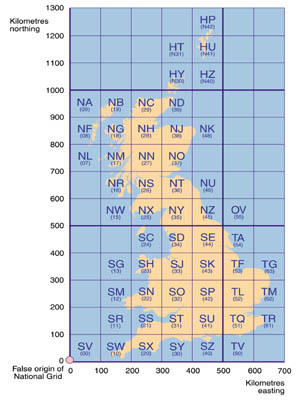Grid Lines/Grid (Kilometre) Squares/Map References

On each Ordnance Survey 1:50000 series map there is a series of intersecting blue Grid Lines; one set runs vertically up and down the map and are known as Eastings, the other horizontally across the map and are known as Northings. Grid Lines are often abbreviated as GL.
Grid Lines are 2cms apart, representing 1 km in real terms.
Grid Lines are consecutively numbered on the outer edge of the map and repeated on the map at intervals of approximately 10 kilometres.
Grid Lines which are multiples of 10, e.g. 10, 20, 30 have a bolder blue line.The Grid Line after 90 is 00 and then the sequence repeats. Each 100 square kilometre area bounded by Eastings of 00 and Northings of 00 has a unique two-letter code. This code is shown in each corner of the map and astride any 00 line at the edge of the map.
The diagram to the left shows the two-letter code coverage for the UK.

Each cell in the grid is known as a Grid Square. A synonymous term is Kilometre Square.
A Grid Square may be referenced by a number formed from the leftmost Easting and lowermost Northing. So a square bounded by Eastings 51 and 52, and Northings 21 and 22, would be referred to as a four-digit numbered Grid Square 5121. An example is square 5121 on map 154 which contains junction 8 of the M11 - or to be precise TL 5121 since it is located in the TL zone, or using the map number as a differentiator 154/5121.

Check this link for the OS 1:50000 Maps coverage of the UK.

Note that the horizontal reference is quoted first. An easy way to remember the order is the phrase "Along the corridor (travel horizontally) then up the stairs (travel vertically)."
Grid Squares are often abbreviated as GS.

A Grid Square defines an area of one kilometre by one kilometre.

To be more precise about a particular point, Grid Squares may be further divided using, at a first level, an imaginary grid formed by 10 vertical and 10 horizontal divisions. We thus extend the numbering of a Grid Square by the addition of an additional digit appended to the Easting and Northing. So generically we now have the capability of defining a point such as 51x21y, where x is the number of tenths (of a kilometre) horizontally and y is the number of tenths (of a kilometre) vertically. This is known as a 6-figure Map Reference - a synonymous term is 6-digit Grid Reference

510210 represents the bottom left corner of our sample square; 510220 the top left; 520210 the bottom right; 520220 the top right; and 515215 the centre of the Grid Square. Note that such references do not actually refer to a point but to a mini-square of 100 metres by 100 metres whose origin is given by the 6-figure reference. However, it is common in rally navigation that a 6-digit reference actually refers to the origin itself; although qualification is often by quoting fractions of tenths (¼, ½, ¾) e.g. 510½214½.
Even greater precision is achieved by extending the reference to 8 or 10 digits. 510½214½ could be written as 51052145 (8-digit); 510¼214¾ could be written as 5102521475 (10-digit).

The Ordnance Survey Fact Sheets Using the National Grid and Map References provide more information.